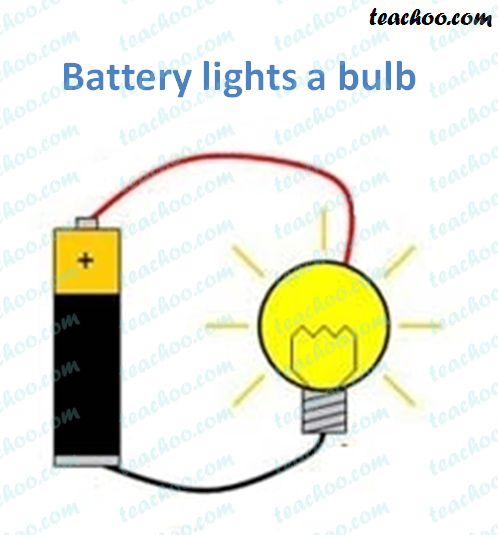NCERT Questions

Class 9
Chapter 11 Class 9 - Work and Energy (Term 2)

## A battery lights a bulb. Describe the energy changes involved in the process.

###(adsbygoogle = window.adsbygoogle || []).push({});

When a battery lights a bulb, first energy transformation takes place in the battery and then in the bulb.

In the battery, the chemical energy gets converted into electrical energy.

Battery: Chemical energy Electrical energy

In the bulb, the electrical energy from the bulb gets converted into heat energy and then into light energy.

Bulb: : Electrical energy Heat energy Light energy.

So, Energy Changes are

Chemical energy Electrical energy Heat energy Light energy